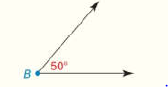Chapter 1.CR, Problem 51CR### Elementary Geometry for College St...

6th Edition
Daniel C. Alexander + 1 other
ISBN: 9781285195698

#### Solutions

Chapter
Section### Elementary Geometry for College St...

6th Edition
Daniel C. Alexander + 1 other
ISBN: 9781285195698
Textbook Problem
1 views

# Given: m ∠ B = 50 ° Construct: An angle whose measure is 20 °To determine

To find:

The complete construction of an angle whose measure is 20°.

Explanation

Given:

The given figure is,

mB=50°.

Approach:

The steps are given below for the complete construction.

a) Draw two circles using the point A and B as center with equal radii AB. Let C be the point of intersection of these circles. Now draw the line segments AC,BC and AB.

b) Draw an angle mA=60°. Bisect this angle to get an angle that measures 30°.

c) A circle is drawn from point A with AD and AE as radius where point D lies on AC and point E lies on AB. Two circles are drawn from point D and E with same radius AD and AE

### Still sussing out bartleby?

Check out a sample textbook solution.

See a sample solution

#### The Solution to Your Study Problems

Bartleby provides explanations to thousands of textbook problems written by our experts, many with advanced degrees!

Get Started

#### Expand each expression in Exercises 122. (x+1x)2

Finite Mathematics and Applied Calculus (MindTap Course List)

#### Find all solutions of the equation. 21. x2 + 2x + 5 = 0

Single Variable Calculus: Early Transcendentals, Volume I

#### True or False: is conservative.

Study Guide for Stewart's Multivariable Calculus, 8th

#### Sometimes, Always, or Never: If {an} is increasing and bounded above, then {an} converges.

Study Guide for Stewart's Single Variable Calculus: Early Transcendentals, 8th